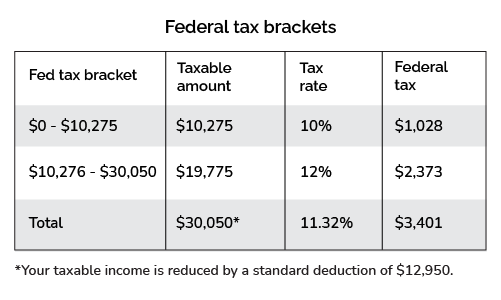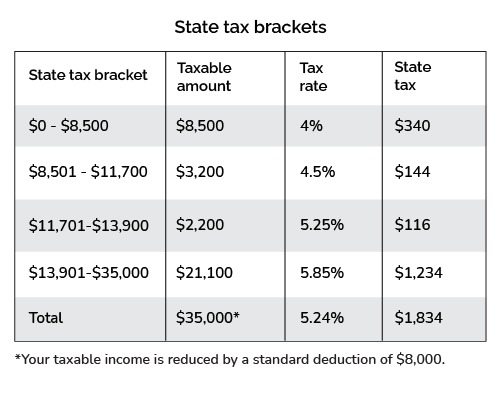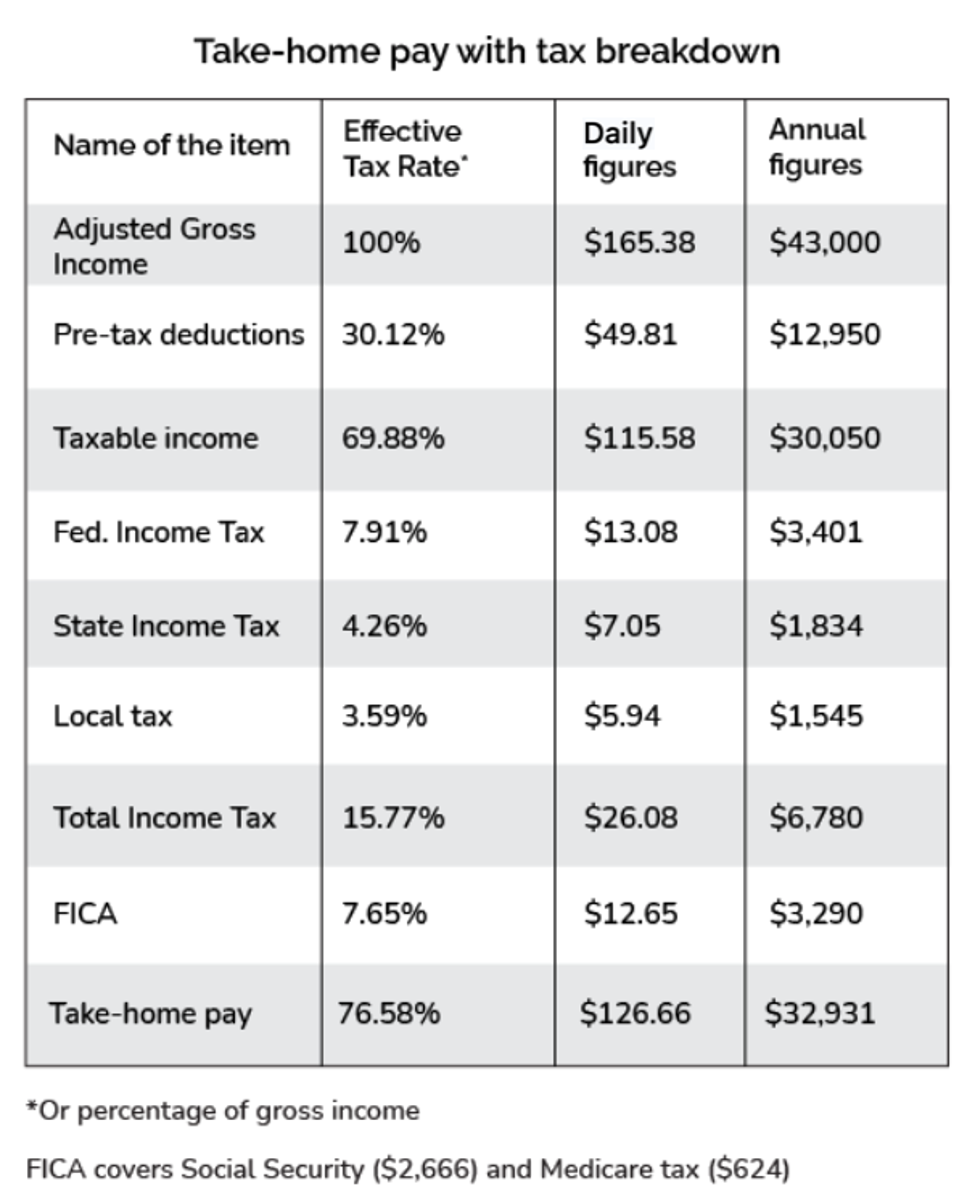# New York Tax Calculator

Created by Tibor Pál, PhD candidate and Arturo Barrantes
Reviewed by Dominik Czernia, PhD and Adena Benn
Based on research by
Tax Foundation
Last updated: Jan 02, 2023

The amazing New York tax calculator helps you calculate how much of your adjusted gross income (AGI) you will devote to taxes during 2022. We know New York is one of the most expensive cities in the world, and the fact that it has extra taxes compared to other states makes it more complicated to calculate. Thus, we have created the New York income tax calculator so you can get a pretty realistic approach to the amount of taxes you will have to pay in April and plan accordingly.

The New York paycheck calculator covers a wide range of topics, including effective tax rates, tax brackets, state taxes, deductions, and even tax credits, so keep reading to find out how to calculate your New York state income tax.

## What taxes are included in the New York tax calculator?

The taxes a New York citizen has to pay include the state income tax, the local income tax, and the general ones: the FICA tax and the federal income tax.

So what is adjusted gross income (AGI):

🙋 AGI is gross income reduced by adjustments such as tuition costs, interest on student loans, alimony payments, and contributions to retirement accounts.

Once we have our AGI, we can proceed with the federal tax rate:

Tax type / Filing status

Single

Married & Jointly

Married & Separately

FICA

7.65%: Social security (6.2%) + medicare (1.45%) taxes

Federal income tax

Progressive tax brackets from 10% to 37%

Deductions in USD

12,950

25,900

12,950

19,400

State income tax

Progressive tax brackets from 4% to 10.90%

Local tax

Progressive tax brackets from 3.078% to 3.876%

The New York tax calculator already includes all the specifics indicated above; however, since we want to make everything crystal clear, we will include the next table: tax brackets for the New York state income tax. You should check the tax bracket calculator in case you need more information about the federal income tax.

The following table is for those who are single and filing in New York for 2022. It also applies to married/registered domestic partners who file separately.

Tax rate

Taxable income bracket

Tax owed*

4%

$0 to$8,500

4% of taxable income

4.5%

$8,501 to$11,700

$340 + 4.5% of the amount over$8500

5.25%

$11,701 to$13,900

$484 + 5.25% of the amount over$11,700

5.85%

$13,901 to$80,650

$600 + 5.85% of the amount over$13,900

6.25%

$80,651 to$215,400

$4,505 + 6.25% of the amount over$80,650

6.85%

$215,401 to$1,077,550

$12,927 + 6.85% of the amount over$215,401

9.65%

$1,077,551 to$5,000,000

$71,984 + 9.65% of the amount over$1,077,550

10.3%

$5,000,001 to$25,000,000

$450,500 + 10.3% of the amount over$5,000,000

10.9%

$25,000,001 or more$2,510,500 + 10.9% over $25,000,000 It is essential to highlight that the single-filing taxpayer has a standard deduction of$8,000 from AGI.

The upcoming table is for those who are married but filing jointly in New York for 2022. It also applies to registered domestic filing jointly and qualified widow(er)s.

Tax rate

Taxable income bracket

Tax owed*

4%

$0 to$17,150

4% of taxable income

4.5%

$17,151 to$23,600

$686 + 4.5% of the amount over$17,150

5.25%

$23,601 to$27,900

$976 + 5.25% of the amount over$23,600

5.85%

$27,901 to$161,550

$1,202 + 5.85% of the amount over$27,900

6.25%

$161,551 to$323,200

$9,021 + 6.25% of the amount over$161,550

6.85%

$323,201 to$2,155,350

$19,124 + 6.85% of the amount over$323,200

9.65%

$2,155,351 to$5,000,000

$144,626 + 9.65% of the amount over$2,155,350

10.3%

$5,000,001 to$25,000,000

$419,135 + 10.3% of the amount over$5,000,000

10.9%

$25,000,001 or more$2,479,135 + 10.9% over $25,000,000 Important to highlight that the married jointly filing taxpayers have a standard deduction of$16,050 from AGI.

Additionally, the amazing New York state tax calculator includes the local state tax, which follows the next configurations:

Married filing jointly

Single or married filing separately

Tax rate

Taxable income bracket

Tax owed

Taxable income bracket

Tax owed

Taxable income bracket

Tax owed

3.078%

$0 to$21,600

3.078% of taxable income

$0 to$12,000

3.078% of taxable income

$0 to$14,400

3.078% of taxable income

3.762%

$21,601 to$45,000

$665 + 3.762% of the amount over$21,600

$12,001 to$25,000

$369 + 3.762% of the excess over$12,000

$14,401 to$30,000

$443 + 3.762% of the excess over$14,400

3.819%

$45,001 to$90,000

$1,545 + 3.819% of the amount over$45,000

$25,001 to$50,000

$858 + 3.819% of the excess over$25,000

$30,001 to$60,000

$858 + 3.819% of the excess over$30,000

3.876%

$90,001 or more$,3264 + 3.876% of the amount over $90,000$50,000 or more

$1,813 + 3.867% of the excess over$50,000

$60,000 or more$2,176 + 3.867% of the excess over $60,000 Lastly, there is a tax exemption of$1,000 if you qualify as a dependent.

## How to use the New York state tax calculator?

Before we start, we need a set of information, including your filing status, as indicated in the New York income tax rate table we shared above. Then:

1. Suppose you are a single filer with an AGI of $43,000 living in New York City and want to know your 2022 total income tax. Let's start with your FICA, which will be: $\small \43,\!000 \times 7.65 \%$. The result is$3,290.

2. Now, it is time for your federal income tax. After considering the 2022 deductions we showed above, your taxable income is $30,050 (that is because $\small \43,\!000 - \12,\!950 = \30,\!050$). Consequently, considering the federal tax progressive income, you pay$1,028 plus 12% of the amount over $10,275, which equals a total federal tax of$3,401.

Let's explain that a bit more: try the New York state tax calculator and in the Results section press Display and select: Add federal income tax brackets. You will see a table like the one below:In that table, you will notice that you reach the 12% bracket, but your income is only partially taxed at that percentage. It is the 11.32% effective tax rate at which your income is evaluated.

1. We continue calculating the New York state income tax. Here you could go ahead and check the other display option: add state income tax brackets.

To learn how to calculate it, follow the next steps:

• Subtract the deductions and exemptions. This means we have an AGI of $\small \43,\!000 - \8,000$, according to the tables above. Consequently, our taxable income is $35,000. • According to the bottom table, since we are in the$13,901-$80,650 bracket, we must pay$600 plus 5.85% of the amount over $13,900 as taxes. In other words, $\small \600 + (\35,\!000-\13,\!901)\times 5.85 \%$ which equals$1,834. See the following table as a summary:1. We need to consider the local tax in the New York paycheck calculator 2022. Similarly to the last step, since we are in the $25,001 to$50,000 bracket, we must pay $858 plus 3.819% of the excess over$25,000 as taxes. That means we owe: $\small \858 + (\43,\!000-\25,\!000)\times 3.819 \%$ which equals $1,545. 2. Finally, we subtract all the taxes: $\small \43,\!000\! -\! \3,\!401\! -\! \1,\!834\! -\! \1,545\! -\! \3,\!290$. The take-home pay amount is$32,931, as you can see in the New York City income tax calculator.In addition, you can estimate your take-home pay for a selected pay frequency – using our tool as a New York paycheck calculator. For example, if you want to see your monthly take-home pay, you need to select monthly pay frequency, resulting in an estimated monthly take-home pay of $2,744.23. ## How to calculate New York income tax for a married couple You can use this Omni Calculator tool New York city income tax calculator or do as follows: 1. Suppose you are married, filing jointly, and making the median income in New York state:$75,000 annually. Subtract 7.65% of the adjusted gross income (AGI). That will go to your FICA: $5,738. 2. Find your marginal tax rate. After deductions ($25,900), we have a marginal tax rate of 12% on $\small \75,\!000 - \25,\!900 = \49,\!100$, but remember, the marginal tax rate is only the last bracket we fit in. In this case, you will be taxed progressively: 10% up to $20,550, equivalent to$2,055, and the rest at 12%. Thus, we must pay $2,055 plus 12% of the amount over$20,550 (the last bracket), or $5,481 in total. Such value represents an effective tax rate of 11.16%. 3. Obtain the New York paycheck tax: Because of your AGI, your standard deduction is$16,050. Hence, your taxable income becomes $58,950. The state income tax is$1,202 plus 5.85% of the amount over $27,900, which equals$3,018.
4. To get the state tax, we verify we fit in the $45,001 to$90,000 bracket. That means we pay $1,545 plus 3.819% of the amount over$45,000, or $2,691 in total. 5. Finally, subtract all the taxes stated above from your AGI to get your take-home pay. By doing that, we obtain an income after tax of$58,072. You can verify the results in the awesome New York state tax calculator.

Finally, if you are considering moving to Alabama, Texas, Illinois, or California, we recommend checking their respective tax calculators:

## FAQ

### Does New York have local income tax?

Yes, New York local income tax includes four brackets: 3.078%, 3.762%, 3.819%, and 3.876% for different income levels and spread in three types of filing status: married filing jointly, single or married filing separately, and head of household.

### Does New York has state income tax?

Yes, New York state income tax includes nine different brackets from 4% to a maximum tax rate of 10.9%. The ranges cover two types of filers: single filing and married filing jointly.

They also include a standard deduction of $8,000 for singles,$16,050 for a couple, and exemptions of $1,000 if you register yourself as a dependent. ### How much tax do I owe if I earn$75,000 in New York?

Assuming you are head of the household, your total income tax is $12,439. Verify it on the New York state income tax calculator from Omni Calculator or: 1. Subtract the pre-tax deduction:$19,400 from AGI. Your taxable income is $55,600; thus, your federal income tax is$6,379. Do not forget FICA (Federal Insurance Contributions Act).
2. Get the state income tax: Subtract a deduction of $11,200 and determine the tax bracket you reach (5.85%). You owe$3,302.
3. Find your local tax bracket: 3.876%. That means $2,757. 4. Sum all values above. Your total income tax is$12,439.

### Are there any New York city tax deductions or tax credits?

The state tax includes a deduction of $8,000 for single filers and$16,050 for married filers. Regarding the local tax, there are no deductions.

Thus, the best way to decrease the tax amount is to reduce what you pay as state tax with tax credits such as the NYC School Tax Credit, the NYC Household Credit, or the NYC Real Property Credit.

## Disclaimer

You should consider the New York tax calculator as a model for financial approximation. All figures and balances are estimates based on the data you provided in the specifications that are not exhaustive, despite our best effort. In addition, the calculator potentially omits information relevant to determining the exact income tax figures.

For these reasons, we created the New York tax calculator for instructional purposes only. Still, if you experience a relevant drawback or encounter any inaccuracy, we are always pleased to receive helpful feedback and advice.

Tibor Pál, PhD candidate and Arturo Barrantes
\$
/year
Pay frequency
Daily
Year of income
2022
Single
Are you a resident in NYC?
Yes
Results
Display...
summary table only
People also viewed…

### Meat footprint

Check out the impact meat has on the environment and your health.

### Real GDP

Use the real GDP calculator (gross domestic product) to convert nominal GDP into real GDP.

### Real interest rate

Real interest rate calculator helps you to find out the real, inflation-adjusted cost of borrowing and the real yield to the lender or to an investor.

### Sunbathing

Do you always remember to put on sunscreen before going outside? Are you sure that you use enough? The Sunbathing Calculator ☀ will tell you when's the time to go back under an umbrella not to suffer from a sunburn!